# A Novel Autoregressive Co-Variance Matrix and Gabor Filter Ensemble Convolutional Neural Network (ARCM-GF-E-CNN) Model for E-Commerce Product Classification

A Novel Autoregressive Co-Variance Matrix and Gabor Filter Ensemble Convolutional Neural Network (ARCM-GF-E-CNN) Model for E-Commerce Product Classification
Department of CSE, Koneru Lakshmaiah Education Foundation, Vaddeswaram, Guntur, Andhra Pradesh, India

Corresponding Author Email:
Page:
163-168
|
DOI:
https://doi.org/10.18280/ria.360119
20 October 2021
|
Accepted:
3 December 2021
|
Published:
28 February 2022
| Citation

OPEN ACCESS

Abstract:

With the advancement of technologies intelligent and automated environments are rapidly evolved with deep learning and transfer learning techniques. However, the existing technique exhibits different difficulties due to increases in processing data complexity. This research developed an Artificial Intelligence (AI) framework for e-commerce product classification. The data for analysis is collected from different website sources and images are classified. The proposed AI framework is stated as Autoregressive Co-Variance Matrix and Gabor Filter Ensemble Convolutional Neural Network (ARCM-GF-E-CNN). The ARCM-GF-E-CNN incorporates an auto-regressive Co-variance matrix for the classification of online product images. The collected database is categorized into class based on features of the image. The simulation results expressed that the proposed ARCM-GF-E-CNN exhibits higher accuracy for the validation and testing dataset. Further, the analysis of ARCM-GF-E-CNN with existing technique expressed that the proposed classifier increases accuracy, precision, recall, and F1-score.

Keywords:

online product, ensemble classifier, autoregressive co-variance matrix and Gabor filter ensemble convolutional neural network (ARCM-GF-E-CNN), pre-processing, artificial intelligence, convolutional neural network (CNN)

1. Introduction

Globally, e-commerce is characterised as an emerging economic component that cannot be overstated in terms of client pleasure. At the moment, e-commerce is more profitable and has higher customer satisfaction . This has an impact on the country's Gross Domestic Product (GDP) (GDP). Shoppers have a more difficult time finding information on e-commerce because of the difficulty associated with information discovery. This requirement for suitable information aids in information discovery. Users look for products online by category or by the name of the product. However, identifying the exact product category is difficult to product identification . Various classification techniques are used in the product categorization for the search category. Based on the assessment of the search method by inputting the name of the product, the product category is tough. Character search, on the other hand, is regarded as challenging for retrieving product names . However, extracting product names from a search product based on the character is difficult. Product identification via image is a straightforward and quick method of retrieving a product .

The designed product of the image is used in online product categorisation. The image product package is connected to logos, items, letters, product manufacturers, and so on. In this case, the product text is made up of several picture characters that take into account the product aesthetics and contents . The online product is used for filtering image attributes with readily available information, showing personal preferences based on user profile, and categorising images based on content. However, classification is seen as a vital problem for e-commerce processing. Classification is used in a variety of applications, including image retrieval, product taxonomy, better scalability, and improved overall accuracy [6, 7]. In e-commerce, product classification is an efficient method for processing. It involved the classification of products related to various merchants to take into account. Text tagging is used in traditional data processing techniques to achieve improved accuracy .

At present, the classification of product is subjected to a vast range of issues that are processed by several applications. The product classification can be of tagging and image-based classification which utilizes a classification model. The presence of high dimensionality feature images content analysis and inhomogeneity is evaluated for evaluation of image classification. Several studies are conducted for image-based classification characteristics . Recently, Artificial Intelligence (AI) is incorporated with the image search technique for product retrieval. In product search technology product classification services are examined for examining packages either through logos or text. Usually, unique product images within online shopping are not as efficient as a packaging product. The images of packages are described with different text product images. This resulted in reduced classification performance in Artificial Intelligence technology .

This paper proposed an Artificial Intelligence-based classifier for texture identification and classification of online product images. The proposed classifier model is represented as Autoregressive Co-Variance Matrix and Gabor Filter Ensemble Convolutional Neural Network (ARCM-GF-E-CNN). The collected online product images are categorized into classes and pre-processing is performed with the Gabor filter. Simulation results expressed that the proposed ARCM-GF-E-CNN exhibits higher accuracy than the existing technique. The comparative analysis stated that the proposed ARCM-GF-E-CNN exhibits improved accuracy, precision, Recall, and F1-score approximately 16%, 10%, 12%, and 15% respectively.

This paper is organized as follows: In section, II represented related works for online product classification. In section III presented about proposed ARCM-GF-E-CNN for classification followed by simulation results comparison in section IV. Finally, in section V overall conclusion and future enhancement is presented.

2. Related Works

Classification is a class of machine learning techniques for the classification of a large amount of data based on consideration of various characteristics. The algorithm which performs classification is defined as a classifier. As per pattern recognition theory, in classification technique feature extraction and recognition are considered as a key strategy. In the classification of e-commerce images, Support vector machine (SVM) and Artificial neural network (ANN) is employed. Several researchers focused on product classification techniques in e-commerce applications. Chen  developed a visual-based image classifier model for the classification of product tagging. It explores consumer product feasibility using image classification. The classification technique adapts Scale Invariant Feature Transform (SIFT) for descriptors of image classification. The developed classification technique provides an accuracy value ranges between 66 - 98%. Jia et al.  proposed a supervised image classifier model for the classification of Image-to-Class distance classification (I2C). The classification is conducted based on the Pyramid Histogram of Words (PHOW).

Wang et al.  constructed a Support Vector Machine (SVM) classifier for the representation of different models. Every level in SVM classifier is trained based on consideration of features and special levels, The SVM classifier likelihood outputs are trained based on Gaussian Radial Basis Function (RBF) kernel. The proposed classifier achieves an accuracy of 86.9% for the classification of the product. The proposed integrated model is stated as Pyramid Histogram of Words (PHOW) descriptor and Image-to-Class distance (PHOW/I2C). In the training phase, local features are illustrated in soft-voting descriptors with PHOW with dense sampling. Yang et al.  developed an unexplored classification for text and image signal with less discriminative analysis for classification. The simulation analysis explored that precision of 100% and recall value of 90%. Chanti and Caplier  presented a two-layer SVM classifier for combining spatial level with pyramid histogram of words (PHOW) and pyramid histogram of orientated gradients (PHOG) descriptors. The experimental analysis demonstrated that six chi-squares kernel-based SVM classifier for training special level. The classification is based on the consideration of the Gaussian RBF kernel. The experimental analysis stated that accuracy is achieved at 79.1%.

The training is based on visual word representation with assigned labels based on consideration of variance and distribution values. The analysis is based on the normalization of query image chi-square distance estimation with specific descriptors. The experimental analysis demonstrated that for 30 product classes classification accuracy is achieved as 84%. Qu et al.  developed a kernel-based SVM classifier with the integration of multiple features. The features considered for analysis are complimentary local descriptors and global descriptors. The developed technique provides improved product based image feature fusion.

Qu et al.  developed an integrated PHOG (Pyramid of Histograms of Orientation Gradients) descriptors for image classification. The developed algorithm process high-dimensional data in Support Vector Machine in a flexible plane with a special layout of image type. The experimental analysis demonstrated that accuracy increases with an increase in training data. Oyewole and Olugbara  developed a framework for color image classification with the integration of SVM kernel with radial basis function (LaRBF). The color features of images are extracted classes from PI100 database with consideration of histogram of oriented gradient (HOG) with consideration five different models such as RGB, RGB, XYZ, HSV, and YUV.

The artificial Neural Network (ANN) power model exhibits a nonlinear relationship between input variables based on the user profile. The data product's large number causes overfitting with ANN integrated with the application of e-commerce. To overcome the overfitting problem several research is considered. Savareh et al.  introduced the cross-validation technique for overcoming overfitting. In the training dataset, associated weights are applied and validated. The testing set is employed in the learning and training process with stopping criteria of the increase in error rate. In Ref.  Pearson et al. conducted object recognition in e-commerce business with the integration of Convolution Neural Network (CNN) and natural language processing (NLP). Simulation analysis exhibited a classification accuracy of 86%. Choi et al.  demonstrated a deep learning technique with a categorization method. In the deep learning model e-commerce website uses Recurrent Neural Network (RNNs) for data attributes feature generation with the classification of generated features.

Ristoski et al.  developed a leverages neural model and deep learning technique through standard classification. The developed approach involved in the management of feature extraction is based on attribute-value pairs with appropriate descriptions. The constructed Deep Convolutional Neural Network (DCNN) is involved in the embedding of product images. Vieira and Moreira  constructed a hierarchical classification technique with a deep learning technique in e-commerce image classification with improved classification accuracy. Hessel Tuinhof et al.  developed two-stage deep learning for classification images. The classification is based on a data-driven approach for automatic extraction of customer available information.

3. Development of Ai Based ARCM-Gf-E-CNN Framework for Online Product Classification

This section demonstrates the process adopted in AI for the classification of online products. The constructed AI model incorporates Autoregressive Co-Variance Matrix and Gabor Filter for classification of images.

a. Data sources and processing

In this research, online product photos are collected from websites such as dreamstime.com and gettyimages.com. The collected dataset images consist of 1,453 and 4,035 image database. The collected images are categorized and placed in appropriate places with continuous annotation and checking with the classification of images for achieving high-quality training datasets. The collected images are digitized with the conversion of color spaces. To improve the accuracy of classification image data were standardized with a deep learning model.

b. Autoregressive Co-Variance Matrix and Gabor Filter (ARCM-GF) for extraction of feature and texture

Feature extraction is a major image processing task in the proposed ARCM-GF-E-CNN framework. Feature extraction is used in the estimation of a collection of components in the estimation of a desire set via background subtraction. The image components are determined using data from the background subtraction. In general, picture characteristics are extracted using the online product image feature extraction. This study employs textural features in conjunction with the representation of Co-occurrence matrix estimation. The extracted texture feature from ARCM is used in the second-arrangement factual approach.

At first, color images are converted in to grey-level intensity pair for estimation of neighbor pixels for estimation of image scale and orientation. The ARCM equation is computed using Eq. (1) as follows:

$G(i,j)=\frac{N(i,j)}{\sum\nolimits_{m=0}^{l-1}{\sum\nolimits_{n=0}^{l-1}{N(m,n)}}}$    (1)

where, the image grey values are represented as i and j with relative recurrence frequency matrix of N(i,j) is estimated using the Eq. (2) stated as follows:

\begin{align} & N(i,j)=num(\{[({{x}_{1}},{{y}_{1}}),({{x}_{2}},{{y}_{2}})]\} \\ & \left| {{x}_{2}}-{{x}_{1}}=d\cos \theta ,{{y}_{2}}-{{y}_{2}} \right.= \\ & d\sin \theta ,I({{x}_{1}},{{y}_{1}})=i,I({{x}_{2}},{{y}_{2}})=j) \\\end{align}     (2)

where, pixel position is represented as (x1, y1) and (x2, y2). The image grey value is denoted as I(.). The certain criteria for matching image pixel is represented as num.

i. Gabor filter for online product feature extraction

Gabor filter is class of linear filter with impulse response estimated in harmonic function estimated using Gaussian function. The Gabor filter involved in localization for segmentation of texture. The input with various texture areas provides output with sub images filter. Gabor filter is based on Gabor Elementary Function (GEF) with decomposition of space. The Gabor filter GEF is evaluated using the Eq. (3):

$h(s,t)=g\left( {{s}^{\grave{\ }}},{{t}^{\grave{\ }}} \right)\exp [j2\Pi \left( {{M}_{s}}+{{N}_{t}} \right)]$     (3)

In above Eq. (3), $\left( {{s}^{\grave{\ }}},{{t}^{\grave{\ }}} \right)=\left( s\cos \theta +t\sin \theta -s\sin \theta +t\cos \theta \right)$ denoted in spatial-domain coordinates. Let coordinates of frequency-domain is denoted as (m, n), the 2D-frequency is denoted as (M,N). The sinusoidal frequency of complex exponents is denoted as 2-D with measured frequency of $F=\sqrt{M^{2}+N^{2}}$ and $\varphi=\tan ^{-1} N / M$ with sinusoidal waveform. The 2-D Gaussian coordinates of function g (x, y) is presented in Eq. (4):

$g(s, t)=\frac{1}{2 \pi \sigma_{s} \sigma_{t}} \exp \left\{-\frac{1}{2} * \frac{s^{2}}{\sigma^{2}}+\frac{t}{}^{2}\right\}$      (4)

where, filter bandwidth and spatial extent is denoted as $\sigma_{s}$ and $\sigma_{t}$. The GEF with Gaussian modulated complex in sinusoidal form is illustrated using Fourier transform h(s, t) is defined in Eq. (5):

$H(m,n)=\exp \left\{ -\frac{1}{2}\left[ \left( {{\sigma }_{s}} \right){{\left[ m-M \right]}^{2}} \right]+{{\left( {{\sigma }_{t}}\left[ n-N \right] \right)}^{2}} \right\}$      (5)

where, $\left[(\mathrm{m}-\mathrm{M})^{\prime},(\mathrm{n}-\mathrm{N})^{\prime}\right]=[(\mathrm{m}-\mathrm{M}) \cos \theta+(\mathrm{n}-\mathrm{N}) \sin \theta,-(\mathrm{m}-\mathrm{M}) \sin \theta+$ $(\mathrm{n}-\mathrm{N}) \cos \theta]$. The gaussian determined is represented as σs, σt with rotation angle of θ with frequency value of (M, N). The GEF reduction ratio is calculated based on the estimation of parameter θ with assumption of σs = σt = σ. The GEF is represented in Eq. (6):

$h(s,t)=\frac{1}{2\pi {{\sigma }_{s}}}\exp \left\{ -\frac{{{s}^{2}}+{{t}^{2}}}{2{{\sigma }^{2}}} \right\}\exp [j2\pi ({{M}_{s}}+{{N}_{t}})]$      (6)

The Gabor filter is represented using $O_{h}$ in Eq. (7) as follows,

$O(s,t)={{O}_{h}}(i(s,t))=\left| i(s,t)\otimes h(s,t) \right|$      (7)

ii. ARCM-GF-E-CNN for product subtraction in online product with Ensemble CNN

In this Ensemble Convolutional Neural Network (E-CNN) is employed after ARCM-GF. The dataset collected from those websites is trained for prediction of class. The classification is based on a multiclass approach with 10 apparel classes employed in online fashion products. The training of each image is the size of 28 x 28 with a total pixel size image of 784 pixels with different label classes.

Based on the training sequence line of codes randomly select input images for evaluation of matched label. Through the estimation of label class and name, images are randomly selected with the visual representation of random images. Based on the selected images values its length and width are evaluated. With estimated values object is the subplot with verification of image class code. It returns object and axes subplot are identified with identification of the different locations. The classes are verified based on the set of codes based on names with random visualization of images. In Figure 1 working architecture for the proposed E-CNN is represented for classification.

## 1.png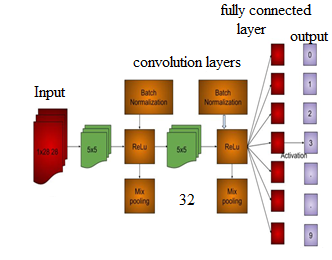Figure 1. Working architecture for E-CNN

In next stage, data is classified as training and testing with visualization of training image data used for ensemble convolutional neural network. The data size is tested with running the model with unfolded data for training, testing and validation. In next step, E-CNN model is utilized for classification of dataset with visualization of model loss and accuracy. The image values are consistent with consideration of training epochs 200 as hyperparameter.

The E-CNN model is tested for prediction of online product classification for produced label class for prediction of output. The model values is predicted based on assigned label class 0 for prediction for prediction of label classes to predict image label class. The Figure 2 provides the incorporated research methodology adopted for ARCM-GF-E-CNN for classification of online products. In Figure 2 research methodology adopted for developed ARCM-GF-E-CNN is presented.

## 2.png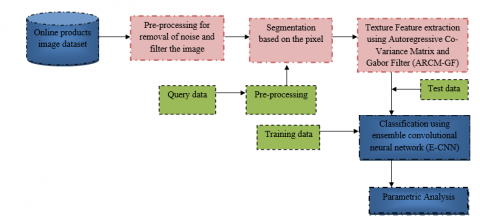Figure 2. Overall flow of ARCM-GF-E-CNN

Initially, the online product database is taken as input followed by pre-processing got noise elimination with Gabor filter along with image resizing. Based on the image intensity images are segmented with extraction of image feature and extracted with Autoregressive Co-Variance Matrix and Gabor Filter (ARCM-GF). Upon completion of feature extraction features are classified as training and testing for feature extraction in E-CNN for conversion of pixel intensity spatial frequency characteristics for texture analysis.

Algorithm for ARCM:

a) Step1: Quantize the image data.

b) Step2: Create the ARCM.

c) Step3: Make the ARCM symmetric:

1. Make a transposed copy of the ARCM
2. Add this copy to the ARCM itself.

d) Step4: Normalize the ARCM.

Calculate the selected Feature. This calculation uses only the values in the ARCM.

4. Simulation Analysis

The proposed ARCM-GF-E-CNN model is evaluated based training and testing database for classification of product online images. The performance is implemented in MATLAB 2019b software for differentiation of training and testing. The performance of proposed classifier is comparatively examined with existing technique such as

The simulation parameters considered for analysis of proposed ARCM-GF-E-CNN model is analyzed with consideration of various performance metrics. The simulation performance metrics considered for analysis are presented as follows:

Accuracy: Accuracy determines the closeness of the detection made by the classifier. It is calculated using the Eq. (8):

$Accuracy=\frac{TP+TN}{TP+FP+FN+TN}$     (8)

Precision: It is defined as ratio of positive values to the total predicted values. It is calculated using Eq. (9):

$\Pr ecision=\frac{TP}{TP+FP}$      (9)

Recall: It is defined as ratio of correctly predicted positive values to the actual observation class. It is measured using Eq. (10):

$\operatorname{Re}call=\frac{TP}{TP+FN}$     (10)

where, TP signifies true positive, TN refers to true negative, FN states the false negative, and FP refers to false positive.

F1-Score: It is stated as ration of weighted average of precision and recall and it is measured using equation (11) as follows:

$F1-Score=2*\frac{\left( \operatorname{Re}call*\Pr ecision \right)}{\left( \operatorname{Re}call+\Pr ecision \right)}$     (11)

The proposed ARCM-GF-E-CNN classifier is evaluated for classification of online products. The cross-validation is set as 10 epochs for classification of online products. In Table 1 accuracy validation of different testing set is presented.

Table 1. Accuracy validation levels

 Training Configuration Validation Set Testing Set 3D-CDCN (RGB only) 53.81 56.12 3D-CDCN (Depth only) 56.35 58.43 3D-CDCN (Fusion) 62.04 66.27 MultiD-CNN (Linear fusion) 69.84 72.53 MultiD-CNN (Max fusion) 66.37 68.24 MultiD-CNN (Product fusion) 67.42 71.16 ARCM-GF-E-CNN 69.56 73.69

## 3.png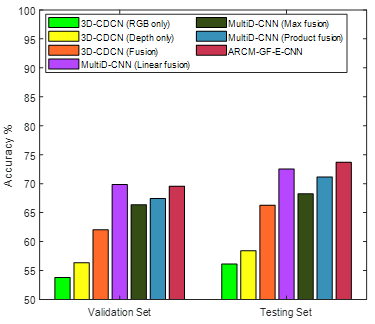Figure 3. Comparison of accuracy

In Figure 3 comparative analysis of validation and testing set are illustrated the comparative analysis exhibited that proposed ARCM-GF-E-CNN exhibits higher accuracy.

The comparative analysis of accuracy estimation for validation set and testing set is presented. The analysis expressed that proposed ARCM-GF-E-CNN exhibits higher accuracy in validation set and testing set also increased. The proposed ARCM-GF-E-CNN exhibits validation set accuracy of 69.56 and testing set accuracy value as 73.69. In Table 2 comparative analysis of proposed ARCM-GF-E-CNN classifier with existing technique is presented.

From Figures 4-6 comparative plot for measured performance metrics is illustrated.

Table 2. Comparative Analysis

 Precision Class 3D-CDCN  (RGB only) 3D-CDCN  (Depth only) 3D-CDCN (Fusion) MultiD-CNN  (Linear fusion) MultiD-CNN (Max fusion) MultiD-CNN  (Product fusion) ARCM-GF-E-CNN Class 0 72 78 80 83 87 84.5 89 Class 1 79 84.6 76 86.7 71 88 90 Class 2 75 81.6 84.2 88 88 89 91 Recall Class 3D-CDCN  (RGB only) 3D-CDCN (Depth only) 3D-CDCN  (Fusion) MultiD-CNN (Linear fusion) MultiD-CNN  (Max fusion) MultiD-CNN  (Product fusion) ARCM-GF-E-CNN Class 0 70 55.6 77 81 86 86 89 Class 1 83 57.8 76 80.6 85.5 84.57 86.8 Class 2 88 51.8 78.1 82.2 88 88 90.2 F1-Score Class 3D-CDCN (RGB only) 3D-CDCN  (Depth only) 3D-CDCN (Fusion) MultiD-CNN (Linear fusion) MultiD-CNN (Max fusion) MultiD-CNN (Product fusion) ARCM-GF-E-CNN Class 0 69.2 63.60 65.7 74.63 81.26 81.64 84.6 Class 1 77.4 67.29 70.83 78.49 88.7 76.9 88.3 Class 2 83.3 69.2 73.6 81.1 86.3 85 90.6

## 4.png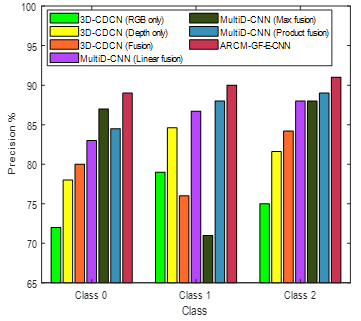Figure 4. Comparison of precision

## 5.png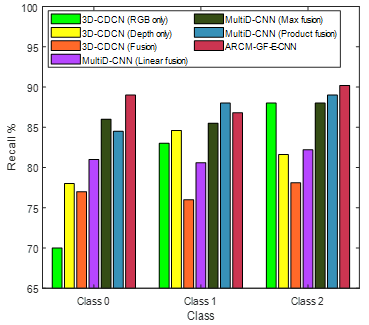Figure 5. Comparison of recall

## 6.png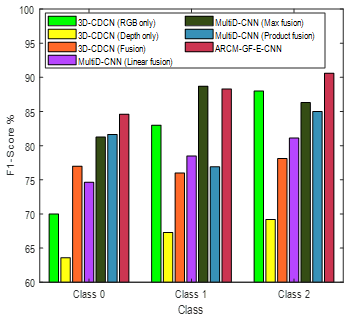Figure 6. Comparison of F1-Score

In above Table 2 performance of proposed ARCM-GF-E-CNN with existing technique is presented. The comparative analysis stated that proposed approach exhibits higher precision, Recall and F1-Score value. The performance of proposed ARCM-GF-E-CNN is approximately 10% higher than the existing technique. In terms of recall and F1-Score proposed ARCM-GF-E-CNN is approximately 12% and 15% higher than existing technique.

5. Conclusion

Online product image classification is subjected to a vast range of challenges in existing techniques. To overcome limitations such as time consumption this research developed an ARCM-GF-E-CNN for classification. The developed technique performs autoregressive with Gabor filtering for online product image classification. Finally, to achieve an improved classification accuracy ensemble classifier is adopted. The comparative analysis stated that the proposed ARCM-GF-E-CNN achieved higher classification accuracy, precision, recall, and F1-score. In the future, the proposed technique will be applied in the classification of medical data based on consideration of classes for reducing processing time.

References

 Singh, A., Tucker, C.S. (2017). A machine learning approach to product review disambiguation based on function, form and behavior classification. Decision Support Systems, 97: 81-91. https://doi.org/10.1016/j.dss.2017.03.007

 Mandhula, T., Pabboju, S., Gugulotu, N. (2019). Predicting the customer’s opinion on amazon products using selective memory architecture-based convolutional neural network. The Journal of Supercomputing, 76: 5923-5947. https://doi.org/10.1007/s11227-019-03081-4

 Maiyar, L.M., Cho, S., Tiwari, M.K., Thoben, K.D., Kiritsis, D. (2019). Optimising online review inspired product attribute classification using the self-learning particle swarm-based Bayesian learning approach. International Journal of Production Research, 57(10): 3099-3120. https://doi.org/10.1080/00207543.2018.1535724

 Salegna, G. (2018). Classification model and e-loyalty implications for online services. International Journal of Quality and Service Sciences, 10(1): 72-83. https://doi.org/10.1108/IJQSS-12-2016-0084

 Wakil, K., Alyari, F., Ghasvari, M., Lesani, Z., Rajabion, L. (2019). A new model for assessing the role of customer behavior history, product classification, and prices on the success of the recommender systems in e-commerce. Kybernetes, 49(5): 1325-1346. https://doi.org/10.1108/K-03-2019-0199

 Yang, Z., Xiong, G., Cao, Z., Li, Y., Huang, L. (2019). A decision method for online purchases considering dynamic information preference based on sentiment orientation classification and discrete DIFWA operators. IEEE Access, 7: 77008-77026. https://doi.org/10.1109/ACCESS.2019.2921403

 Li, G., Li, N. (2019). Customs classification for cross-border e-commerce based on text-image adaptive convolutional neural network. Electronic Commerce Research, 19(4): 779-800. https://doi.org/10.1007/s10660-019-09334-x

 Mandhula, T., Pabboju, S., Gugulotu, N. (2019). Predicting the customer’s opinion on amazon products using selective memory architecture-based convolutional neural network. The Journal of Supercomputing, 76: 5923-5947. https://doi.org/10.1007/s11227-019-03081-4

 Lee, Y., Chung, M., Cho, S., Choi, J. (2019). Extraction of product evaluation factors with a convolutional neural network and transfer learning. Neural Processing Letters, 50(1): 149-164. https://doi.org/10.1007/s11063-018-9964-8

 Wu, J., Cai, Y., Huang, Q., Xu, J., Wong, R.C.W., Chen, J. (2020). Two-level convolutional neural network for aspect extraction. In International Conference on Database Systems for Advanced Applications, pp. 93-105. Springer, Cham. https://doi.org/10.1007/978-3-030-59413-8_8

 Chen, K. (2017). Part-based deep representation for product tagging and search. In Second International Workshop on Pattern Recognition, 104431D. https://doi.org/10.1117/12.2280300

 Jia, S.J., Wang, P., Jia, P.Y., Hu, S.P. (2017). Research on data augmentation for image classification based on convolution neural networks. In 2017 Chinese Automation Congress (CAC), pp. 4165-4170. https://doi.org/10.1109/CAC.2017.8243510

 Wang, Z., Wang, S., Zhang, P., Li, H., Zhong, W., Li, J. (2019). Weakly supervised fine-grained image classification via correlation-guided discriminative learning. In Proceedings of the 27th ACM International Conference on Multimedia, pp. 1851-1860. https://doi.org/10.1145/3343031.3350976

 Yang, J., Kannan, A., Batra, D., Parikh, D. (2017). LR-GAN: Layered recursive generative adversarial networks for image generation. arXiv preprint arXiv:1703.01560.

 Chanti, D.A., Caplier, A. (2018). Improving bag-of-visual-words towards effective facial expressive image classification. Proceedings of the 13th International Joint Conference on Computer Vision, Imaging and Computer Graphics Theory and Applications, 5: 145-152. https://doi.org/10.5220/0006537601450152

 Qu, Y., Fang, B., Zhang, W., et al. (2018). Product-based neural networks for user response prediction over multi-field categorical data. ACM Transactions on Information Systems (TOIS), 37(1): 1-35. https://doi.org/10.1145/3233770

 Oyewole, S.A., Olugbara, O.O. (2018). Product image classification using Eigen Colour feature with ensemble machine learning. Egyptian Informatics Journal, 19(2), 83-100. https://doi.org/10.1016/j.eij.2017.10.002

 Savareh, B.A., Bashiri, A., Behmanesh, A., Meftahi, G.H., Hatef, B. (2018). Performance comparison of machine learning techniques in sleep scoring based on wavelet features and neighboring component analysis. PeerJ, 6: e5247. https://doi.org/10.7717/peerj.5247

 Pearson, K.D., Nelson, G., Aronson, M.F., et al. (2020). Machine learning using digitized herbarium specimens to advance phenological research. BioScience, 70(7): 610-620. https://doi.org/10.1093/biosci/biaa044

 Choi, S., Ha, H., Hwang, U., Kim, C., Ha, J.W., Yoon, S. (2018). Reinforcement learning based recommender system using biclustering technique. arXiv preprint arXiv:1801.05532.

 Ristoski, P., Petrovski, P., Mika, P., Paulheim, H. (2018). A machine learning approach for product matching and categorization. Semantic Web, 9(5): 707-728. https://doi.org/10.3233/SW-180300

 Vieira, M.G., Moreira, J. (2017). Classification of e-commerce-related images using hierarchical classification with deep neural networks. In 2017 Workshop of Computer Vision (WVC), pp. 114-119. https://doi.org/10.1109/WVC.2017.00027

 Tuinhof, H., Pirker, C., Haltmeier, M. (2018). Image-based fashion product recommendation with deep learning. In International Conference on Machine Learning, Optimization, and Data Science, pp. 472-481. Springer, Cham. https://doi.org/10.1007/978-3-030-13709-0_40